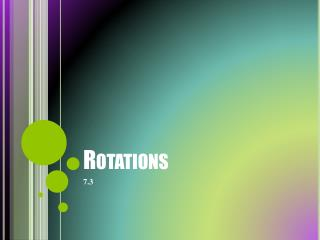# Rotations - PowerPoint PPT PresentationDownload PresentationRotations

RotationsDownload Presentation## Rotations

- - - - - - - - - - - - - - - - - - - - - - - - - - - E N D - - - - - - - - - - - - - - - - - - - - - - - - - - -
##### Presentation Transcript

1. Rotations 7.3

2. How do rotations work? • Every point rotates around the center in a circle. • Since the radius of the circle will not change, the point is just as far from the center as it was, just in a new direction.

3. Vocabulary • Rotation: • A type of transformation in which a figure is turned about a fixed point. • Center of Rotation: • The fixed point of a rotation is called the center of rotation. • Angle of Rotation: • The angle formed when rays are drawn from the center of rotation to a point and its image.

4. When Rotating around the origin… • Every 90˚ rotation will exchange the x and y coordinates. • Every 90˚ rotation will change the quadrant the figure is in.

5. Types of Rotations • Rotation about vertex of figure • Rotation about a point not on figure • Rotation about a point inside the figure.

6. Double Reflections… Activity Time! • Write your name in one of the triangles, filling the triangle as much as possible! Bubble letters, etc… be creative! “Name Art Rotation”

7. Double Reflections • A double reflection is the same as a rotation! • The angle of rotation is twice the measure of the angle between the lines of reflection

8. What you will be asked to do • 5. CD • 6. FG • 7. EF • 8. AB . • 9. HPA • 10. GPH

9. What you will be asked to do • 14.) A’ (1,3) B’(3,3) C’(3,-1) D’(1,-1) • 15.) A’ (0,2) B’(2,3) C’(4,2) D’(2,1)

10. What you will be asked to do • 11.) 64 • 12.) 64

11. Homework: Finish your “Name Art Rotation” It must be FULLY colored… no white space! 7.3 Pg. 416 13-19, 25-27, 30-35